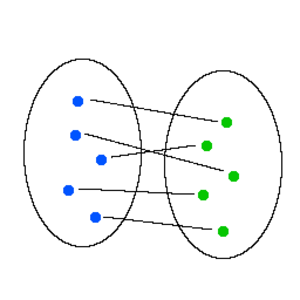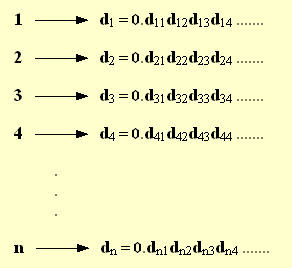## Monday, April 13, 2009

### Counting to InfinityIf you read yesterday's blog, you were probably intrigued, confused, if not angry all at the same time. How can there be different sizes of infinity???? Infinity is infinitely big, right? It's not even a number. It's more of an idea (like love) or perhaps a never-ending destination (I hope you have enough gas).In fact, there ARE different sizes of infinity, some of which are infinitely larger than others. Some are the same size, even though they seemingly have "more" things in them. That's what make mathematics, especially calculus, so exciting and fun (terms used loosely and relatively).

Because the set of numbers known as the Naturals, Wholes, Integers, and Rationals can be arranged into a one-way list by which we can check them off without fear (imagined or real) of skipping anyone, and because a one-to-one correspondence can be established between items on the list in each set, we say they are all infinitely large, yet countable, sets with the samecardinality , or number of elements in them, as the Natural numbers, a theorem known as Dedekind's Theorem. These sets belong to the same "equivalence class." Mathematicians care not whether or now we will ever be able to finish the actual task of counting them, but rather that the process of counting can even take place.

That brings us to today's question: Is the set of Real Numbers, the largest set of non-imaginary numbers, infinitely countable or infinitely uncountable??????

Well Georg Cantor stunned the world when he proved, yes PROVED, that the Real Numbers are NOT countable. How does one go about proving such a thing??? It requires more than just a string of failed attempts at trying to count them. It requires a little creative thought that creates a beautiful poem of irrefutable logic. Cantor proved it by what is know as "Proof by contradiction," which involves presupposing something to be true, then showing that the original assumption leads to a false statement or a conclusion that contradicts, hence the name, the original supposition. This then means that the original assumption is, in fact, false.

His ingenious proof followed these lines of logic:

Cantor started by assuming the Reals ARE countable, kind of like the American Justice system of "innocent until proven guilty," except for number sets. Which if so, they can be put in a one-t0-one correspondence with the Natural numbers, so we can list them in the same one-way order as the Naturals. This is demonstrated in the diagram at left. Now we can use this list to construct a Real number, call it x that varies from every number in our list in at least one decimal place. We will require that this x will differ from the n-th digit in the list in the n-th decimal place. So x = x1x2x3 . . . such that x1 does not equal d11, x2 does not equal d22, x3 does not equal d33, etc. This is know as Cantor's diagonalization and does not rely on any real decimal expansions.

Now, x is a decimal number, and x is also less than one, so it must be in our list. But where? It can't be first, since x's first digit differs from d1's first digit. It can't be second in the list, because x and d2 have different hundredths place digits. In general, x is not equal to dn, since their nth digits are not the same. We have just created a value of x that is NOT on our original list!!!!! Now we have a contradiction!!!!!!Remember that our list was SUPPOSED to contain ALL of the Real numbers, which has to include our fabricated value of x, which obviously wasn't originally there!! Does that make sense?

Therefore, our assumptions were false, and a one-to-one correspondence with the Naturals must NOT be possible, ergo, the Reals are NOT countable.

The consequence of this means that the Reals are infinitely more infinite than the Natural numbers!

Put another way, there are just as many numbers on the number line between "0" and "1" as there are on the ENTIRE number line!!!!!This startling consequence is actually how Cantor established his proof. The decimals listed in the example above are just a list of decimals limited between zero and one. However, no generality is lost if we use this interval, because if this subset of the real numbers in uncountable, then the full set is uncountable too!So now we've established infinite countable sets, and infinite uncountable sets which are infinitely larger than the countable variety. But what about infinite sets of truly different (infinite) sizes?

Enter the term "Power Sets." Sounding very similar to what I call my Bicep workout sessions, a mathematical Power Set is the set of all subsets of any given set. Yes, sets themselves can be elements of larger sets that contain them. Confused yet?

I'll address this tomorrow!